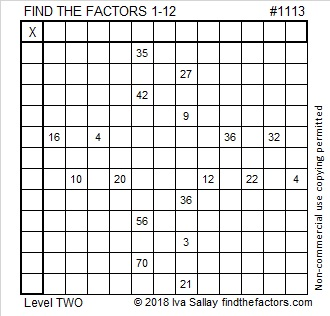# 1113 and Level 2

If you’ve never solved a Find the Factors puzzle before, this level 2 puzzle will be a good one to try.  Just make sure each number 1 to 12 is written in the top row and the first column and that those numbers and the clues in the puzzle form a multiplication table. You can fill in the rest of the table later or not at all. Have fun!Print the puzzles or type the solution in this excel file: 12 factors 1111-1119

Here is some information about the number 1113:

• 1113 is a composite number.
• Prime factorization: 1113 = 3 × 7 × 53
• The exponents in the prime factorization are 1, 1, and 1. Adding one to each and multiplying we get (1 + 1)(1 + 1)(1 + 1) = 2 × 2 × 2 = 8. Therefore 1113 has exactly 8 factors.
• Factors of 1113: 1, 3, 7, 21, 53, 159, 371, 1113
• Factor pairs: 1113 = 1 × 1113, 3 × 371, 7 × 159, or 21 × 53
• 1113 has no square factors that allow its square root to be simplified. √1113 ≈ 33.361651113 is the hypotenuse of a Pythagorean triple:
588-945-1113 which is 21 times (28-45-53)

1113 is made with three consecutive digits in these two consecutive bases:
It’s 789 in BASE 12 because 7(144) + 8(12) + 9(1) = 1113, and
it’s 678 in BASE 13 because 6(169) + 7(13) + 8(1) = 1113